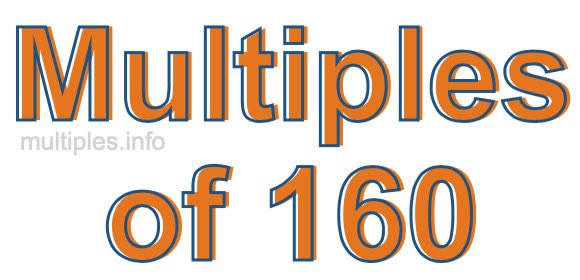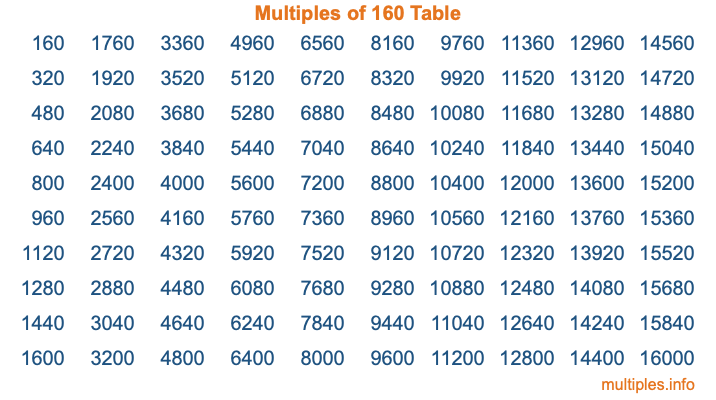Multiples of 160Welcome to the Multiples of 160 page. Here we will first teach you everything you will ever need to know about the multiples of 160, and then give you a study guide summary of everything we taught you to make sure you remember it all. Use this page to look up facts and learn information about the multiples of 160. This page will make you a multiples of one hundred sixty expert!

Definition of Multiples of 160
Multiples of 160 are all the numbers that when divided by 160 equal an integer. Each of the multiples of 160 are called a multiple. A multiple of 160 is created by multiplying 160 by an integer.

Therefore, to create a list of multiples of 160, you start with 1 multiplied by 160, then 2 multiplied by 160, then 3 multiplied by 160, and so on for as long as you want. Thus, the list of the first five multiples of 160 is 160, 320, 480, 640, and 800. To see a larger list of multiples of 160, see the printable image of Multiples of 160 further down on this page. We also have a category where you can choose any nth multiple of 160.

Multiples of 160 Checker
The Multiples of 160 Checker below checks to see if any number of your choice is a multiple of 160. In other words, it checks to see if there is any number (integer) that when multiplied by 160 will equal your number. To do that, we divide your number by 160. If the the quotient is an integer, then your number is a multiple of 160.

Is  a multiple of 160?

Least Common Multiple of 160 and ...
A Least Common Multiple (LCM) is the lowest multiple that two or more numbers have in common. This is also called the smallest common multiple or lowest common multiple and is useful to know when you are adding our subtracting fractions. Enter one or more numbers below (160 is already entered) to find the LCM.

Check out our LCM Calculator if you need more details about the Least Common Multiple or if you need the LCM for different numbers for adding and subtraction fractions.

nth Multiple of 160
As we stated above, 160 is the first multiple of 160, 320 is the second multiple of 160, 480 is the third multiple of 160, and so on. Enter a number below to find the nth multiple of 160.

th multiple of 160

Multiples of 160 vs Factors of 160
160 is a multiple of 160 and a factor of 160, but that is where the similarities end. All postive multiples of 160 are 160 or greater than 160. All positive factors of 160 are 160 or less than 160.

Below is the beginning list of multiples of 160 and the factors of 160 so you can compare:

Multiples of 160: 160, 320, 480, 640, 800, etc.

Factors of 160: 1, 2, 4, 5, 8, 10, 16, 20, 32, 40, 80, 160

As you can see, the multiples of 160 are all the numbers that you can divide by 160 to get a whole number. The factors of 160, on the other hand, are all the whole numbers that you can multiply by another whole number to get 160.

It's also interesting to note that if a number (x) is a factor of 160, then 160 will also be a multiple of that number (x).

Multiples of 160 vs Divisors of 160
The divisors of 160 are all the integers that 160 can be divided by evenly. Below is a list of the divisors of 160.

Divisors of 160: 1, 2, 4, 5, 8, 10, 16, 20, 32, 40, 80, 160

The interesting thing to note here is that if you take any multiple of 160 and divide it by a divisor of 160, you will see that the quotient is an integer.

Multiples of 160 Table
Below is an image of the first 100 multiples of 160 in a table. The table is in chronological order, column by column. The first column has the first ten multiples of 160, the second column has the next ten multiples of 160, and so on.The Multiples of 160 Table is also referred to as the 160 Times Table or Times Table of 160. You are welcome to print out our table for your studies.

Negative Multiples of 160
Although not often discussed or needed in math, it is worth mentioning that you can make a list of negative multiples of 160 by multiplying 160 by -1, then by -2, then by -3, and so on, to get the following list of negative multiples of 160:

-160, -320, -480, -640, -800, etc.

Multiples of 160 Summary
Below is a summary of important Multiples of 160 facts that we have discussed on this page. To retain the knowledge on this page, we recommend that you read through the summary and explain to yourself or a study partner why they hold true.

There are an infinite number of multiples of 160.

A multiple of 160 divided by 160 will equal a whole number.

160 divided by a factor of 160 equals a divisor of 160.

The nth multiple of 160 is n times 160.

The largest factor of 160 is equal to the first positive multiple of 160.

160 is a multiple of every factor of 160.

160 is a multiple of 160.

A multiple of 160 divided by a divisor of 160 equals an integer.

160 divided by a divisor of 160 equals a factor of 160.

Any integer times 160 will equal a multiple of 160.

Multiples of a Number
Here you can get the multiples of another number, all with the same attention to detail as we did for multiples of 160 on this page.

Multiples of
Multiples of 161
Did you find our page about multiples of one hundred sixty educational? Do you want more knowledge? Check out the multiples of the next number on our list!